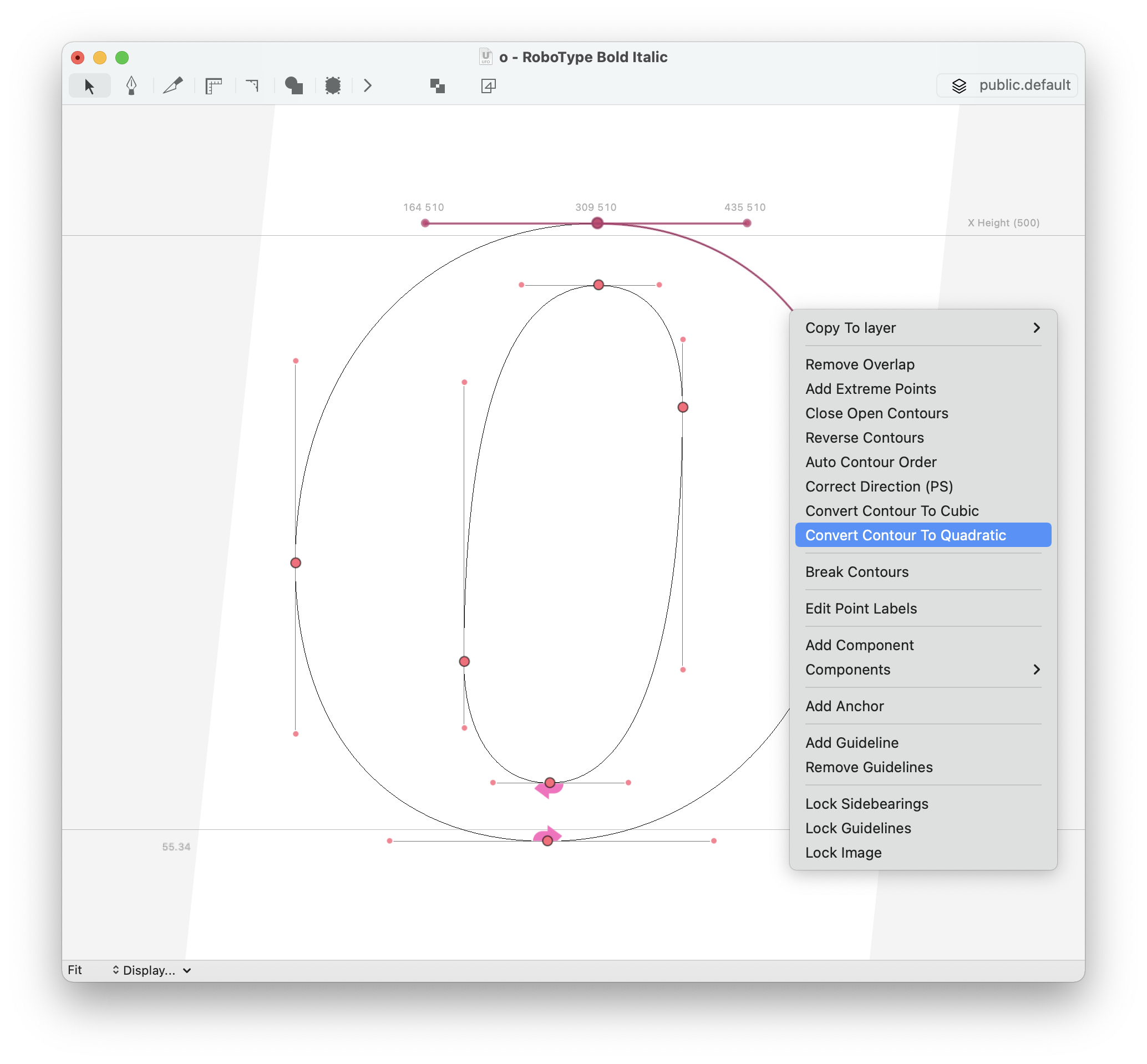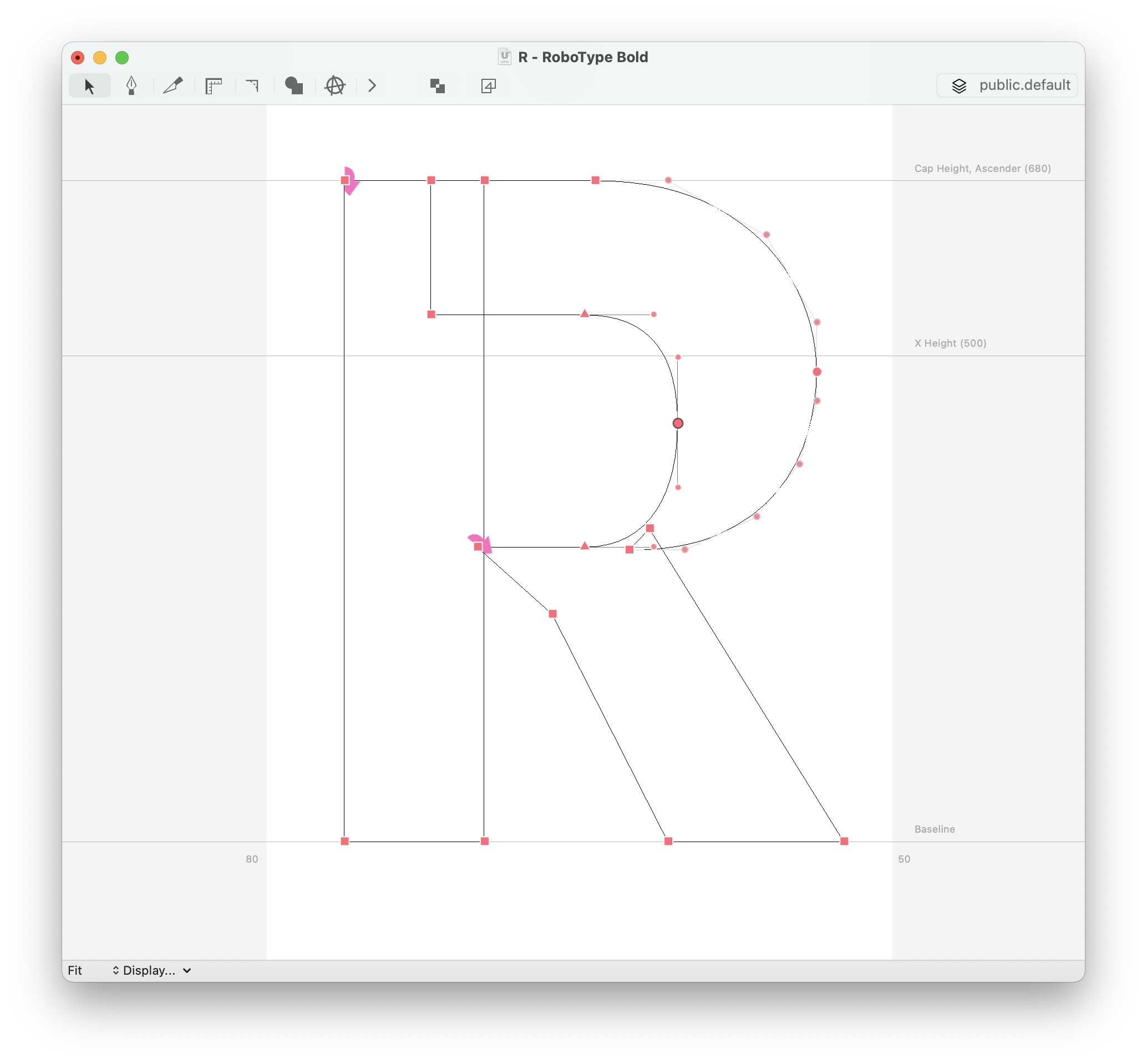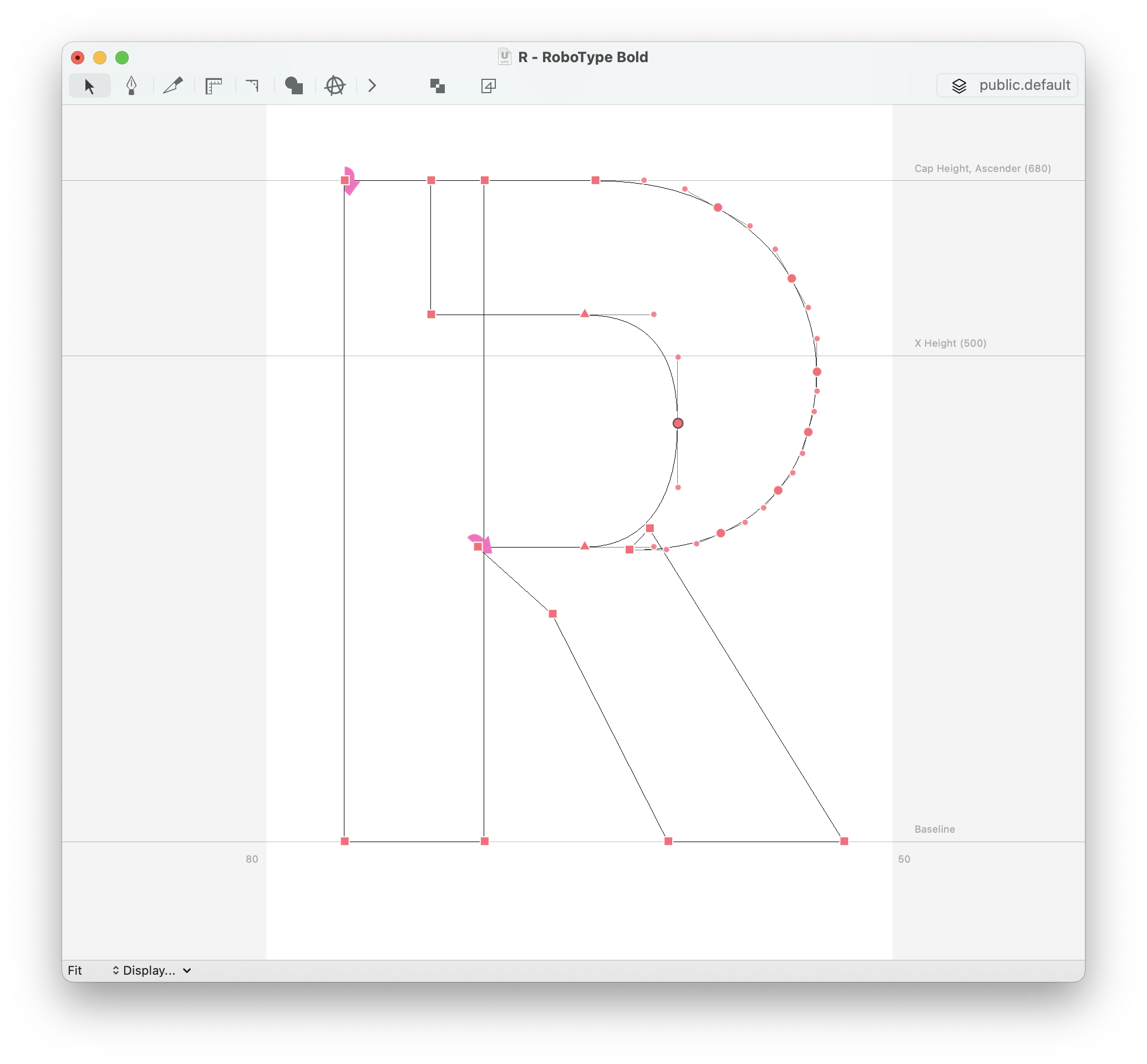## Converting to and from quadratic using the glyph editorNo consistent back and forth translation is possible between quadratics and cubic beziers. So, if you convert from cubic to quadratic, then back to cubic, you’ll never get the same point structure. So, keep that in mind while using this feature.

Under the hood, RoboFont tries to convert the cubic bezier to quadratic maintaining the same point structure (two on-curve points, two off-curve points). If the result is not satisfying, it converts the cubic bezier using an algorithm that does not preserve the point structure.

## Converting to and from quadratic using a script

The script below converts all glyphs in the current (cubic) font to quadratic and then back to cubic.

The conversion is made using RoboFont’s cubic-to-quadratic algorithm, which adds an implied on-curve point and splits each curve segment in two.

``````# check the slideshow for results
font = CurrentFont()
font['R'].convertToCubic()

``````
•Original outline

••